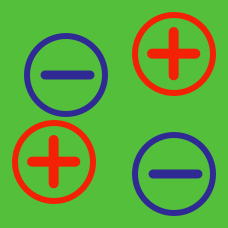Electricity and Magnetism

# Charges and Their Interactions: Level 1-2 Challenges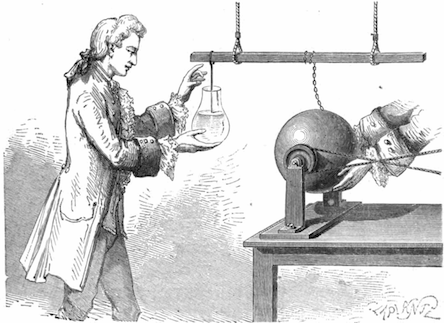When a glass rod is rubbed with a silk cloth, a positive charge is gained by the glass rod. This can be described in terms of the gain or loss of electrons or protons.

Which of the following statements are correct?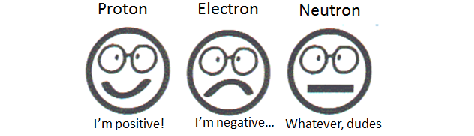In our universe, protons have a positive charge while electrons have a negative charge. If all the charges in the universe were reversed so that electrons had a positive charge and protons had a negative charge, then what would happen to electromagnetic phenomena such radio waves, currents, static electricity, and formation of atoms?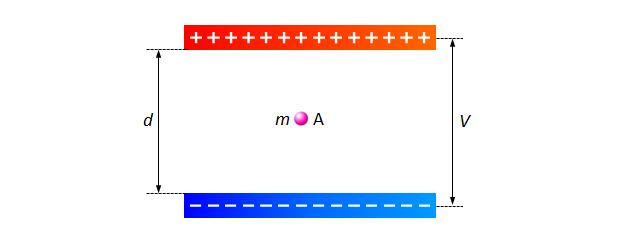As shown above, a charged particle $A$ with mass $m$ is placed between two parallel charged plates parallel to the ground with the negatively charged plate closer to the ground. The distance between the two plates is $d$ and the electric potential difference between the two plates is $V$. If the particle is stationary, then what is the magnitude of the quantity of the electric charge of $A?$

Note: $g$ denotes the gravitational acceleration.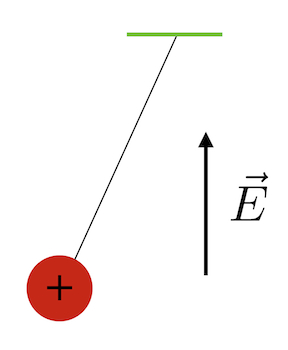Let the period of an uncharged pendulum be $t$. This pendulum is now charged with a charge of $+q$ and placed in a uniform electric field pointing vertically upward. If the new period of the pendulum is denoted by $T,$ which of the following is correct?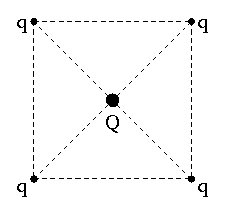It is required to hold four equal point charges $+q$ each in equilibrium at the corners of a square. Find the charge in Coulombs, $Q$, that will do this if kept at the center of the square.


Details and Assumptions:

• $q=1\text{ C}.$
×